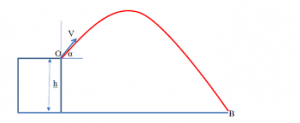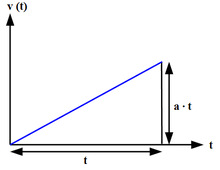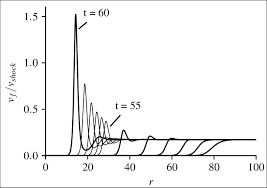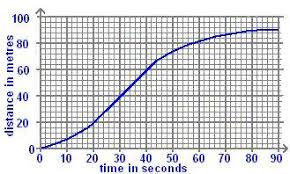## How to Calculate and Solve for Final Velocity | MotionThe image above represents final velocity.

To compute for final velocity, three essential parameters are needed and these parameters are initial velocity (u), acceleration (a) and time (t).

The formula for calculating final velocity:

v = u + at

Where;

v = Final Velocity
u = Initial Velocity
a = Acceleration
t = Time

Let’s solve an example;
Find the Final velocity when the initial velocity is 12, acceleration is 9 and the time is 24.

This implies that;

u = Initial Velocity = 12
a = Acceleration = 9
t = Time = 24

v = u + at
v = 12 + (9 x 24)
v = 12 + 216
v = 228

Therefore, the final velocity is 228 m/s.

Calculating the Initial Velocity when the Final Velocity, the Acceleration and the Time is Given.

u = v – at

Where;

u = Initial Velocity
v = Final Velocity
a = Acceleration
t = Time

Let’s solve an example;
Find the Initial Velocity when the Final Velocity is 32, the acceleration is 12 and the time is 2.

This implies that;

v = Final Velocity = 32
a = Acceleration = 12
t = Time = 2

u = v – at
u = 32 – (12 x 2)
u = 32 – 24
u = 8

Therefore, the initial velocity is 8 m/s.

## How to Calculate and Solve for Acceleration | MotionThe image above represents acceleration.

To compute for acceleration, three essential parameters are needed and these parameters are initial velocity (u), final velocity (v) and time (t).

The formula for calculating acceleration:

a = (v – u) / t

Where;

a = Acceleration
v = Final Velocity
u = Initial Velocity
t = Time

Let’s solve an example;
Find the acceleration when the final velocity is 21, the initial velocity is 10 and time is 8.

This implies that;

v = Final Velocity =21
u = Initial Velocity = 10
t = Time = 8

a = (v – u) / t
a = (21 – 10) / 8
a = 11 / 8
a = 1.375

Therefore, the acceleration is 1.375 m/s².

Calculating the Initial Velocity when the Acceleration, the Final Velocity and the Time is Given.

u = v – at

Where;

u = Initial Velocity
a = Acceleration
v = Final Velocity
t = Time

Let’s solve an example;
Find the initial velocity when the acceleration is 14, the final velocity is 34 and the time is 2.

This implies that;

a = Acceleration = 14
v = Final Velocity = 34
t = Time = 2

u = v – at
u = 34 – (14 x 2)
u = 34 – 28
u = 6

Therefore, the initial velocity is 6 m/s.

## How to Calculate and Solve for Final Velocity | MotionThe image above represents final velocity.

To compute for final velocity, three essential parameters are needed and these parameters are initial velocity (u), acceleration (a) and distance covered (S).

The formula for calculating final velocity:

v = √(u² + 2as)

Where;

v = Final Velocity
u = Initial Velocity
a = Acceleration
s = Distance Covered

Let’s solve an example;
Find the final velocity when the initial velocity is 13, acceleration is 9.8 and the distance covered is 32.

This implies that;

u = Initial Velocity = 13
a = Acceleration = 9.8
s = Distance Covered = 32

v = √(u² + 2as)
v = √(13² + (2 x 9.8 x 32))
v = √(169 + (627.2))
v = √(796.2)
v = 28.21

Therefore, the final velocity is 28.21 m/s.

Calculating the Initial Velocity when the Final Velocity, the Acceleration and the Distance Covered is Given.

u = √v² – 2as

Where;

u = Initial Velocity
v = Final Velocity
a = Acceleration
s = Distance Covered

Let’s solve an example;
Find the initial velocity when the final velocity is 30, the acceleration is 8 and the distance covered is 11.

This implies that;

v = Final Velocity = 30
a = Acceleration = 8
s = Distance Covered = 11

u = √v² – 2as
u = √30² – 2(8 x 11)
u = √900 – 2(88)
u = √900 – 176
u = √724
u = 26.90

Therefore, the initial velocity is 26.90 m/s.

## How to Calculate and Solve for Distance Covered | MotionThe image above represents distance covered.

To compute for distance covered, three essential parameters are needed and these parameters are final velocity (v), initial velocity (u) and time (t).

The formula for calculating distance covered:

S = ((u + v) / 2) t

Where;

S = Distance Covered
u = Initial Velocity
v = Final Velocity
t = Time

Let’s solve an example;
Find the distance covered when the initial velocity is 6, final velocity is 11 and time is 14.

This implies that;

u = Initial Velocity = 6
v = Final Velocity = 11
t = Time = 14

S = ((u + v) / 2) t
S = ((6 + 11) / 2) 14
S = ((17) / 2) 14
S = (8.5) 14
S = 119

Therefore, the distance covered is 119 m.

Calculating the Initial Velocity when the Distance Covered, the Final Velocity and the Time is Given.

u = (2S / t) – v

Where;

u = Initial Velocity
S = Distance Covered
v = Final Velocity
t = Time

Let’s solve an example;
Find the initial velocity when the distance covered is 48, the final velocity is 20 and the time is 4.

This implies that;

S = Distance Covered = 48
v = Final Velocity = 20
t = Time = 4

u = (2S / t) – v
u = (2(48) / 4) – 20
u = (96 / 4) – 20
u = 24 – 20
u = 4

Therefore, the initial velocity is 4 m/s.